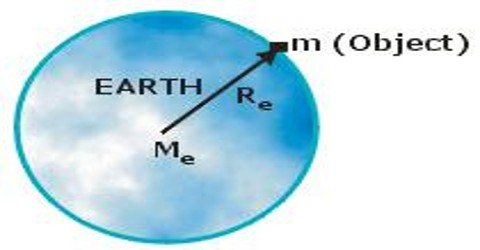Physics

# Acceleration due to GravityAcceleration due to gravity

According to Newton’s laws of motion (1st, 2nd and 3rd), it force is applied on a body acceleration is generated. Gravitation as also a force, this force will create acceleration by acting on a body. So, the acceleration that is produced on a body by gravitational force is called acceleration due to gravity or the rate at which velocity of a freely falling body increases due to the gravitational attraction at a point is called the acceleration due to gravity of that place. It as denoted by ‘g’. It is known from experiment that all bodies from a particular place and in a path having no resistance fall towards the centre of the earth with uniform acceleration. This acceleration varies with the variation of position. So, acceleration due to gravity is independent of body but not independence of position.

Its unit in M.K.S and S.I. system is metre/sec2 (ms-2) and its dimension = [LT2]